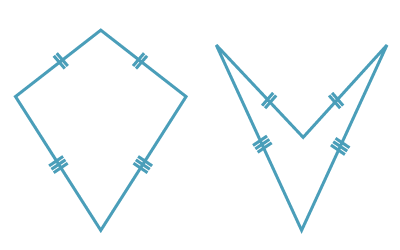### Kites#### Definition of a kite

• A kite is a quadrilateral with two pairs of adjacent sides equal.
• A kite may be convex or non-convex.

#### Properties of a kite

• The opposite sides of a rhombus are parallel.
• The opposite angles of a rhombus are equal.
• The diagonals of a rhombus bisect each vertex angle.
• The diagonals of a rhombus bisect each other at right angles.

#### Test for a kite

A quadrilateral is a kite if:

• one diagonal bisects the vertex angles through which it passes, or
• one diagonal is the perpendicular bisector of the other diagonal.